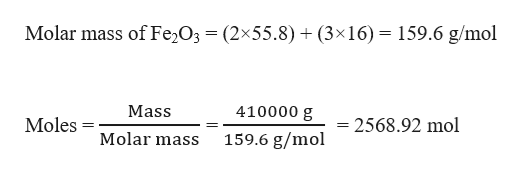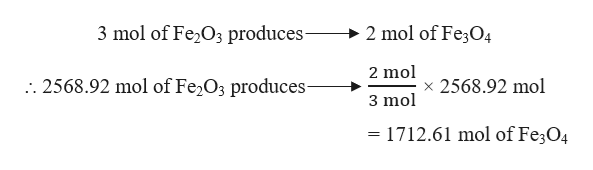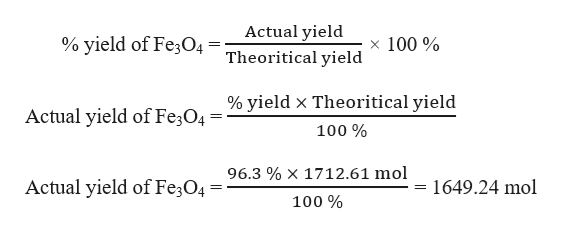A three-step process for producing molten iron metal from Fe2O3 is:3Fe2O3 + CO → 2Fe3O4 + CO2Fe3O4 + CO → 3FeO + CO2FeO + CO → Fe + CO2Assuming that the reactant CO is present in excess and that the yields, respectively, for the three steps are 96.3%, 58.9% and 82.6%, what mass of iron metal would be produced from 410. kg of Fe2O3?

Question

A three-step process for producing molten iron metal from Fe2O3 is:

1. 3Fe2O3 + CO → 2Fe3O4 + CO2
2. Fe3O4 + CO → 3FeO + CO2
3. FeO + CO → Fe + CO2

Assuming that the reactant CO is present in excess and that the yields, respectively, for the three steps are 96.3%, 58.9% and 82.6%, what mass of iron metal would be produced from 410. kg of Fe2O3?

Step 1

Given,

The three-step process for producing molten iron metal from Fe2O3 is:

1. 3 Fe2O3 + CO → 2 Fe3O4 + CO2
2. Fe3O4 + CO → 3 FeO + CO2
3. FeO + CO → Fe + CO2

Since CO2 is gas, so the yields are given for iron compounds only.

% yield of Fe3O4 = 96.3 %

% yield of FeO = 58.9 %

% yield of Fe = 82.6 %

Mass of Fe2O3 = 410 kg = 410000 g

Moles of Fe2O3 can be calculated as:help_outlineImage TranscriptioncloseMolar mass of Fe O3 (2x55.8) (3x16)= 159.6 g/mol Mass 410000 g Moles = 2568.92 mol Molar mass 159.6 g/mol fullscreen
Step 2

In step 1, amount of Fe3O4 produced from Fe2O3 can be calculated as:help_outlineImage Transcriptionclose3 mol of Fe2O3 produces- 2 mol of Fe304 2 mol . 2568.92 mol of Fe2O3 produces- x 2568.92 mol 3 mol = 1712.61 mol of Fe3O4 fullscreen
Step 3

Actual yield of Fe3O4 can be cal...help_outlineImage TranscriptioncloseActual yield Theoritical yield % yield of Fe3O4 x 100% % yield x Theoritical yield Actual yield of Fe3O4' 100% 96.3 %X 1712.61 mol Actual yield of Fe3O4 = 1649.24 mol 100% fullscreen

Want to see the full answer?

See Solution

Want to see this answer and more?

Our solutions are written by experts, many with advanced degrees, and available 24/7

See Solution
Tagged in

Chemistry